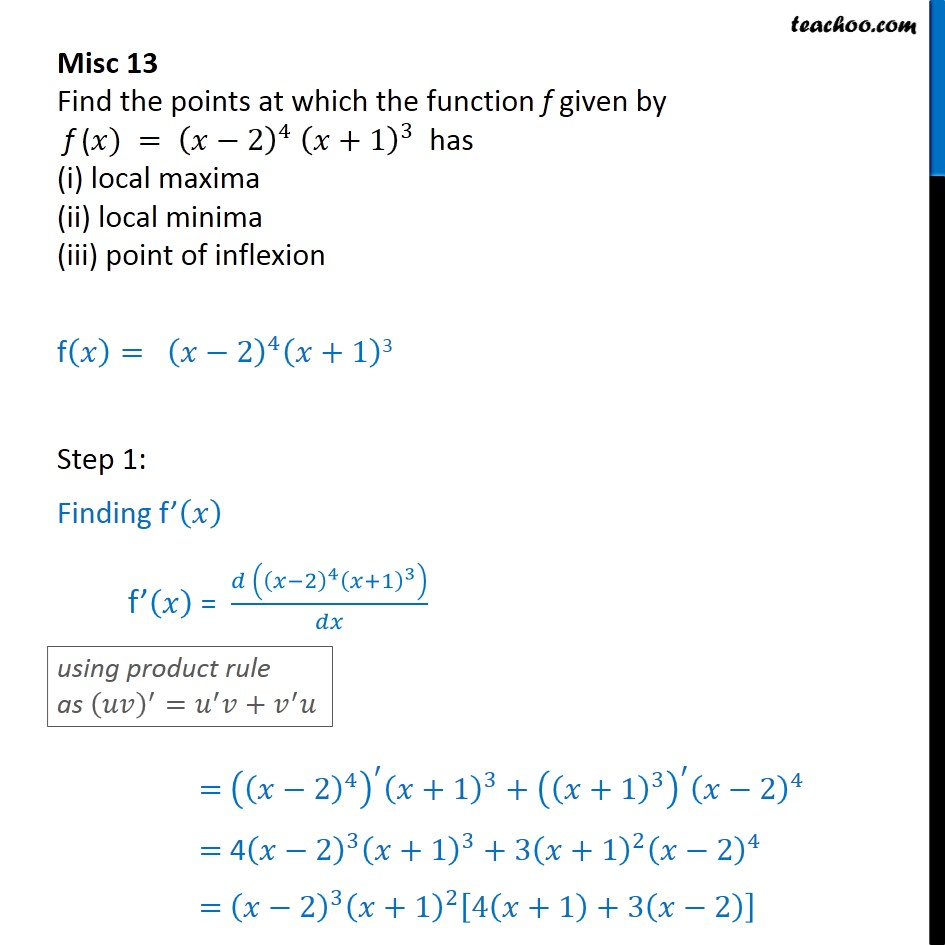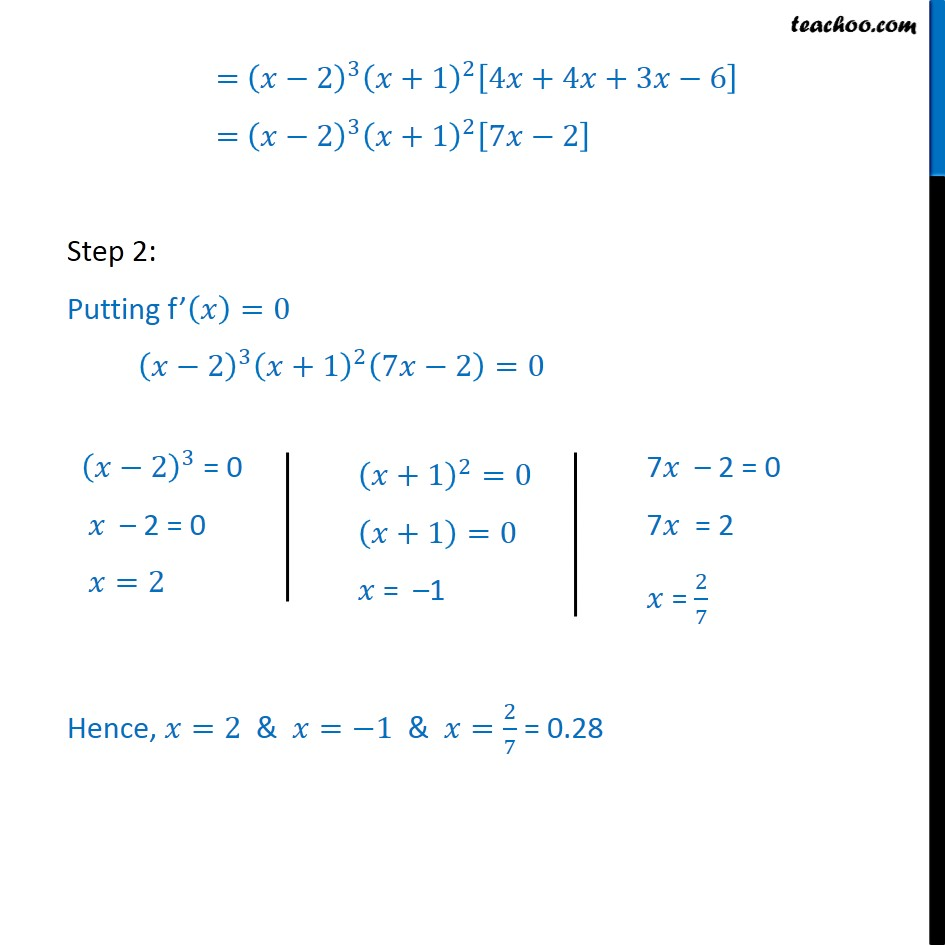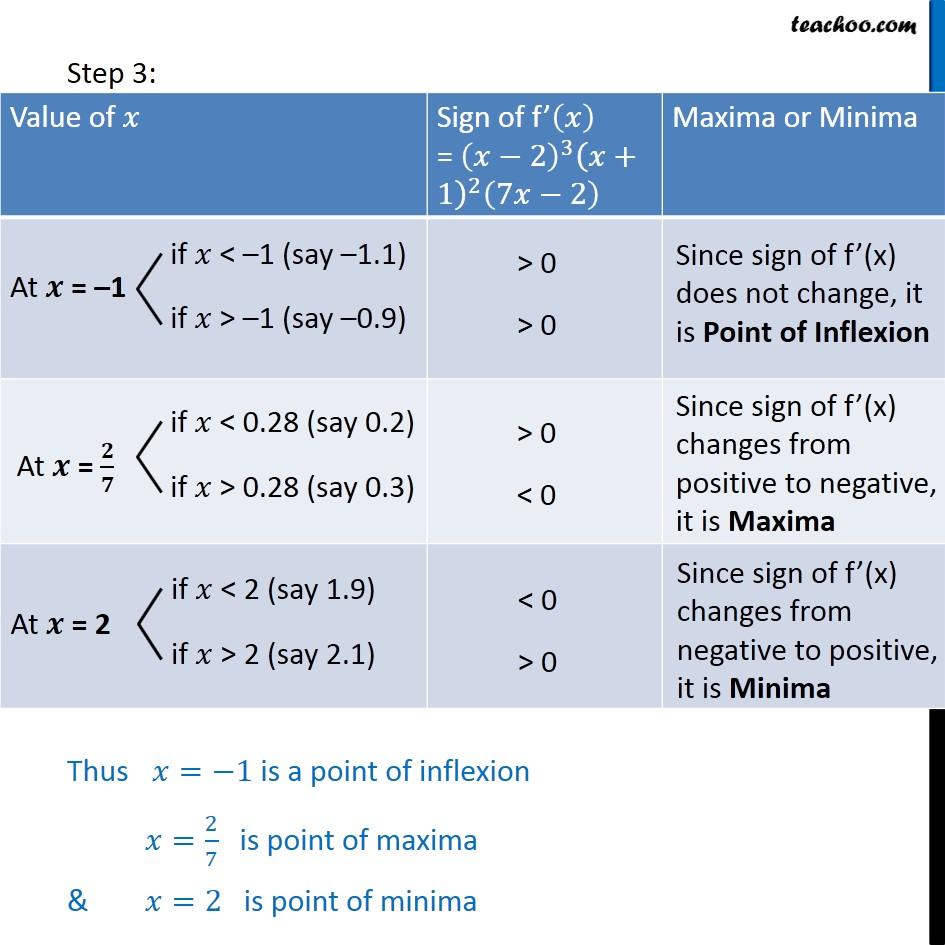1. Class 12
2. Important Question for exams Class 12
3. Chapter 6 Class 12 Application of Derivatives

Transcript

Misc 13 Find the points at which the function f given by f (𝑥) = (𝑥−2)^4 (𝑥+1)^3 has (i) local maxima (ii) local minima (iii) point of inflexionf(𝑥)= (𝑥−2)^4 (𝑥+1)3 Step 1: Finding f’(𝑥) f’(𝑥) = (𝑑 ((𝑥−2)^4 (𝑥+1)^3 ))/𝑑𝑥 using product rule as (𝑢𝑣)^′=𝑢^′ 𝑣+𝑣^′ 𝑢 = 〖((𝑥−2)^4 )^′ (𝑥+1)〗^3+((𝑥+1)^3 )^′ (𝑥−2)^4 = 4(𝑥−2)^3 (𝑥+1)^3+3(𝑥+1)^2 (𝑥−2)^4 = (𝑥−2)^3 (𝑥+1)^2 [4(𝑥+1)+3(𝑥−2)] = (𝑥−2)^3 (𝑥+1)^2 [4𝑥+4𝑥+3𝑥−6] = (𝑥−2)^3 (𝑥+1)^2 [7𝑥−2] Step 2: Putting f’(𝑥)=0 (𝑥−2)^3 (𝑥+1)^2 (7𝑥−2)=0 (𝑥−2)^3 = 0 𝑥 – 2 = 0 𝑥=2 (𝑥+1)^2=0 (𝑥+1)=0 𝑥 = –1 7𝑥 – 2 = 0 7𝑥 = 2 𝑥 = 2/7 Hence, 𝑥=2 & 𝑥=−1 & 𝑥=2/7 = 0.28 Thus 𝑥=−1 is a point of inflexion 𝑥=2/7 is point of maxima & 𝑥=2 is point of minima

Chapter 6 Class 12 Application of Derivatives

Class 12
Important Question for exams Class 12